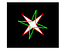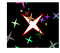In this tutorial, I’ll walk you through how one can scale and rotate a contour based on OpenCV Python API

Follow along, if you want to understand what’s the simple idea behind the implementation.

# Why?

Why would one want to scale or rotate a contour of objects in an image? For me, I had a very similar problem as this StackOverflow question.

I had to scale down the output of an Image Segmentation deep learning model for a specific class, to tightly fit the object of that class. Basically a post processing step for my deep learning model.

I am not able to think of any other “generic” applications for this, may be you can comment for which applications you found it useful.

Plus, I did not find any Python articles regarding this, so I thought I’ll give it a try.

## Learning by example

Throughout this short tutorial, I would be using a simple image to demonstrate my points, below is some code for imports and loading the image, and generating the contours. You know, that standard stuff!

`import cv2import numpy as npimport matplotlib.pyplot as plt# Reading the imageim = cv2.imread('sample_img.png')# Converting image to grayscaleimgray = cv2.cvtColor(im, cv2.COLOR_BGR2GRAY)# Thresholding and getting contours from the imageret, thresh = cv2.threshold(imgray, 127, 255, 0)contours, hierarchy = cv2.findContours(thresh, cv2.RETR_TREE, cv2.CHAIN_APPROX_SIMPLE)im_copy = im.copy()cv2.drawContours(im_copy, contours, 0, (255, 0, 0), 3)plt.imshow(im_copy)plt.axis("off");`

## How to resize contours

Well, the idea is pretty simple, and if you have some understanding of high-school math, below steps would seem very sensible:

• Step 1: Translate the contour to the origin
• Step 2: Scale each point of the contour
• Step 3: Translate back the contour to it’s original place.

Below I explain and show code equivalents of each step.

## Translate the contour to the origin

To translate the contour to the origin, we just have to subtract the coordinates of the centroid of the contour with all the points. And the centroid of the contour can be found using the `cv2.moments` function.

• Getting the centroid of the contour
`M = cv2.moments(cnt)cx = int(M['m10']/M['m00'])cy = int(M['m01']/M['m00'])`
• Translating the contour by subtracting the center with all the points
`cnt_norm = cnt - [cx, cy]`

## Scale each point of the contour

• To scale each point of the contour, we just have to multiply the scale with the contour points.
`cnt_scaled = cnt_norm * scale`

## Translate back the contour to it’s original place.

• This step can be achieved by simply adding back the centroid co-ordinates to the contour points and that’s it!
`cnt_scaled = cnt_scaled + [cx, cy]cnt_scaled = cnt_scaled.astype(np.int32)`

## Combining all the steps into a function for scaling

Hence we can write a simple function based on the above steps as below:

`def scale_contour(cnt, scale):    M = cv2.moments(cnt)    cx = int(M['m10']/M['m00'])    cy = int(M['m01']/M['m00'])    cnt_norm = cnt - [cx, cy]    cnt_scaled = cnt_norm * scale    cnt_scaled = cnt_scaled + [cx, cy]    cnt_scaled = cnt_scaled.astype(np.int32)    return cnt_scaled`

And we can call this function to test that it works fine!

• RED BOUNDRY: Original contour
• GREEN BOUNDRY: Scaled contour
`cnt_scaled = scale_contour(contours, 0.3)im_copy = im.copy()cv2.drawContours(im_copy, contours, 0, (255, 0, 0), 3)cv2.drawContours(im_copy, [cnt_scaled], 0, (0, 255, 0), 3)plt.imshow(im_copy)plt.axis("off");`

## How to rotate contours

Rotating contours are also simple, and again would just take some high school math to understand the steps.

• Step 1: Translate the contour to the origin
• Step 2: Rotate each point of the contour
• Step 3: Translate back the contour to it’s original place.

## Translate the contour to the origin

• Getting the center
`M = cv2.moments(cnt)cx = int(M[‘m10’]/M[‘m00’])cy = int(M[‘m01’]/M[‘m00’])`
• Translating the contour by subtracting the center with all the points
`cnt_norm = cnt — [cx, cy]`

## Rotating each point of the contour

Once you have put the contour to the origin, we have to rotate each point. And for me understanding rotation is much easier in polar co-ordinates as compared to Cartesian co-ordinates (I hope for you too 😉). So, that’s what we’ll do! Convert the points to polar co-ordinates, add the rotation, and convert it back to Cartesian co-ordinates.

• These are some helper functions which I borrowed from here to convert back and forth between Polar and Cartesian co-ordinates
`def cart2pol(x, y):    theta = np.arctan2(y, x)    rho = np.hypot(x, y)    return theta, rhodef pol2cart(theta, rho):    x = rho * np.cos(theta)    y = rho * np.sin(theta)    return x, y`
• Converting the `x-y`(cartesian) co-ordinates to `theta-rho` (polar)
`coordinates = cnt_norm[:, 0, :]xs, ys = coordinates[:, 0], coordinates[:, 1]thetas, rhos = cart2pol(xs, ys)`
• Adding the `angle` to the `thetas`.
`thetas_deg = np.rad2deg(thetas)thetas_new_deg = (thetas_deg + angle) % 360thetas_new = np.deg2rad(thetas_new_deg)`

Note: I converted the radians to degrees because I chose to give `angle` in degrees form (and it makes modulo operation much cleaner). Then I again convert it back to radians.

• Convert the new polar coordinates to cartesian co-ordinates:
`xs, ys = pol2cart(thetas, rhos)cnt_norm[:, 0, 0] = xscnt_norm[:, 0, 1] = ys`

## Translate back the contour to it’s original place.

Add center coordinates to the contours’ coordinates

`cnt_rotated = cnt_norm + [cx, cy]cnt_rotated = cnt_rotated.astype(np.int32)`

## Combining all the steps into a function for rotation

Hence we can write a simple function based on the above steps as below:

`def cart2pol(x, y):    theta = np.arctan2(y, x)    rho = np.hypot(x, y)    return theta, rhodef pol2cart(theta, rho):    x = rho * np.cos(theta)    y = rho * np.sin(theta)    return x, ydef rotate_contour(cnt, angle):    M = cv2.moments(cnt)    cx = int(M['m10']/M['m00'])    cy = int(M['m01']/M['m00'])    cnt_norm = cnt - [cx, cy]        coordinates = cnt_norm[:, 0, :]    xs, ys = coordinates[:, 0], coordinates[:, 1]    thetas, rhos = cart2pol(xs, ys)        thetas = np.rad2deg(thetas)    thetas = (thetas + angle) % 360    thetas = np.deg2rad(thetas)        xs, ys = pol2cart(thetas, rhos)        cnt_norm[:, 0, 0] = xs    cnt_norm[:, 0, 1] = ys    cnt_rotated = cnt_norm + [cx, cy]    cnt_rotated = cnt_rotated.astype(np.int32)    return cnt_rotated`

And we can call this function to test that it works fine!

• RED BOUNDRY: Original contour
• GREEN BOUNDRY: Scaled contour
`cnt_rotated = rotate_contour(contours, 60)im_copy = im.copy()cv2.drawContours(im_copy, contours, 0, (255, 0, 0), 3)cv2.drawContours(im_copy, [cnt_rotated], 0, (0, 255, 0), 3)plt.imshow(im_copy)plt.axis("off");`Original (red) and rotated (green) contour

## Having fun with random rotation, scale, and translation

Here is a simple code to generated random rotation, scaling and translation (not covered in the tutorial but it’s just a simple addition of co-ordinates).

`im_copy = im.copy()cv2.drawContours(im_copy, contours, 0, (255, 0, 0), 3)for i in range(20):    cnt_rotated = rotate_contour(contours, np.random.random() * 90)    cnt_scaled = scale_contour(cnt_rotated, np.random.random() * 0.5)        cnt_translated = cnt_scaled + np.random.randint(low=-200, high=200, size=(2,))        r, g, b = [int(np.random.choice(range(255))) for _ in range(3)]    cv2.drawContours(im_copy, [cnt_translated], 0, (r, g, b), -1)plt.imshow(im_copy)plt.axis("off");`Random rotation + scaling + translation

In-case someone wants to dissect this code, these are the key things I did:

• Generate multiple random contours with a loop. (I know right :D )
• Get randomness with `np.random.random()` which returns random numbers between `[0, 1)`. We can multiply it with desired max value to get values between `[0, max_vale)`.
• For rotation, I chose angle between `0` and `90` degrees, as the image is symmetric at `90` degrees rotation.
• For scale I took random values between `0` to `0.5`, i.e. from 0% to 50% of original contour.
• Randomly translated between `(-200, 200)` across x and y axis.
• Random color generation of rgb values.
• Just drawing the contours with `thickness=-1` which dentes to fill the contour.

# Conclusion

So, as you can see contour scaling and rotation were very easy to implement if you have some basic idea of co-ordinate systems. I hope the tutorial is helpful for you.

Here is the GitHub Gist for the functions for easier usability:

By the way this is my first ever article ever written, 😄 so please do provide any feedback you have in the comments, or you can mail me at nvs232@gmail.com . I would be very grateful for that. 🙏

The jupyter notebook and other material for this tutorial can be found in this GitHub Repository: https://github.com/nvs-abhilash/tutorials/tree/master/tutorials/opencv_contour_scale_rotate

Written by

## Analytics Vidhya

#### Analytics Vidhya is a community of Analytics and Data Science professionals. We are building the next-gen data science ecosystem https://www.analyticsvidhya.com

Welcome to a place where words matter. On Medium, smart voices and original ideas take center stage - with no ads in sight. Watch
Follow all the topics you care about, and we’ll deliver the best stories for you to your homepage and inbox. Explore
Get unlimited access to the best stories on Medium — and support writers while you’re at it. Just \$5/month. Upgrade